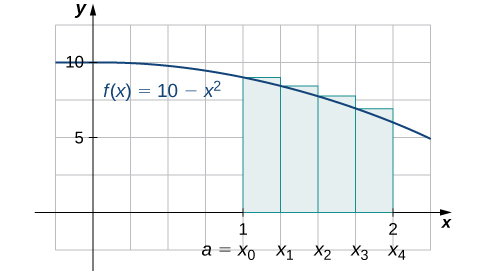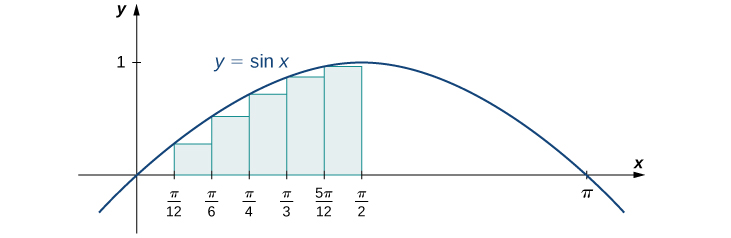# 5.1 Approximating areas  (Page 7/17)

 Page 7 / 17
1. Find an upper sum for $f\left(x\right)=10-{x}^{2}$ on $\left[1,2\right];$ let $n=4.$
2. Sketch the approximation.
1. $\text{Upper sum}=8.0313.$

2.## Finding lower and upper sums for $f\left(x\right)=\text{sin}\phantom{\rule{0.1em}{0ex}}x$

Find a lower sum for $f\left(x\right)=\text{sin}\phantom{\rule{0.1em}{0ex}}x$ over the interval $\left[a,b\right]=\left[0,\frac{\pi }{2}\right];$ let $n=6.$

Let’s first look at the graph in [link] to get a better idea of the area of interest.The graph of y = sin x is divided into six regions: Δ x = π / 2 6 = π 12 .

The intervals are $\left[0,\frac{\pi }{12}\right],\left[\frac{\pi }{12},\frac{\pi }{6}\right],\left[\frac{\pi }{6},\frac{\pi }{4}\right],\left[\frac{\pi }{4},\frac{\pi }{3}\right],\left[\frac{\pi }{3},\frac{5\pi }{12}\right],$ and $\left[\frac{5\pi }{12},\frac{\pi }{2}\right].$ Note that $f\left(x\right)=\text{sin}\phantom{\rule{0.1em}{0ex}}x$ is increasing on the interval $\left[0,\frac{\pi }{2}\right],$ so a left-endpoint approximation gives us the lower sum. A left-endpoint approximation is the Riemann sum $\sum _{i=0}^{5}\text{sin}\phantom{\rule{0.1em}{0ex}}{x}_{i}\left(\frac{\pi }{12}\right).$ We have

$\begin{array}{cc}A\hfill & \approx \text{sin}\left(0\right)\left(\frac{\pi }{12}\right)+\text{sin}\left(\frac{\pi }{12}\right)\left(\frac{\pi }{12}\right)+\text{sin}\left(\frac{\pi }{6}\right)\left(\frac{\pi }{12}\right)+\text{sin}\left(\frac{\pi }{4}\right)\left(\frac{\pi }{12}\right)+\text{sin}\left(\frac{\pi }{3}\right)\left(\frac{\pi }{12}\right)+\text{sin}\left(\frac{5\pi }{12}\right)\left(\frac{\pi }{12}\right)\hfill \\ & =0.863.\hfill \end{array}$

Using the function $f\left(x\right)=\text{sin}\phantom{\rule{0.1em}{0ex}}x$ over the interval $\left[0,\frac{\pi }{2}\right],$ find an upper sum; let $n=6.$

$A\approx 1.125$

## Key concepts

• The use of sigma (summation) notation of the form $\sum _{i=1}^{n}{a}_{i}$ is useful for expressing long sums of values in compact form.
• For a continuous function defined over an interval $\left[a,b\right],$ the process of dividing the interval into n equal parts, extending a rectangle to the graph of the function, calculating the areas of the series of rectangles, and then summing the areas yields an approximation of the area of that region.
• The width of each rectangle is $\text{Δ}x=\frac{b-a}{n}.$
• Riemann sums are expressions of the form $\sum _{i=1}^{n}f\left({x}_{i}^{*}\right)\text{Δ}x,$ and can be used to estimate the area under the curve $y=f\left(x\right).$ Left- and right-endpoint approximations are special kinds of Riemann sums where the values of $\left\{{x}_{i}^{*}\right\}$ are chosen to be the left or right endpoints of the subintervals, respectively.
• Riemann sums allow for much flexibility in choosing the set of points $\left\{{x}_{i}^{*}\right\}$ at which the function is evaluated, often with an eye to obtaining a lower sum or an upper sum.

## Key equations

• Properties of Sigma Notation
$\sum _{i=1}^{n}c=nc$
$\sum _{i=1}^{n}c{a}_{i}=c\sum _{i=1}^{n}{a}_{i}$
$\sum _{i=1}^{n}\left({a}_{i}+{b}_{i}\right)=\sum _{i=1}^{n}{a}_{i}+\sum _{i=1}^{n}{b}_{i}$
$\sum _{i=1}^{n}\left({a}_{i}-{b}_{i}\right)=\sum _{i=1}^{n}{a}_{i}-\sum _{i=1}^{n}{b}_{i}$
$\sum _{i=1}^{n}{a}_{i}=\sum _{i=1}^{m}{a}_{i}+\sum _{i=m+1}^{n}{a}_{i}$
• Sums and Powers of Integers
$\sum _{i=1}^{n}i=1+2+\text{⋯}+n=\frac{n\left(n+1\right)}{2}$
$\sum _{i=1}^{n}{i}^{2}={1}^{2}+{2}^{2}+\text{⋯}+{n}^{2}=\frac{n\left(n+1\right)\left(2n+1\right)}{6}$
$\sum _{i=0}^{n}{i}^{3}={1}^{3}+{2}^{3}+\text{⋯}+{n}^{3}=\frac{{n}^{2}{\left(n+1\right)}^{2}}{4}$
• Left-Endpoint Approximation
$A\approx {L}_{n}=f\left({x}_{0}\right)\text{Δ}x+f\left({x}_{1}\right)\text{Δ}x+\text{⋯}+f\left({x}_{n-1}\right)\text{Δ}x=\sum _{i=1}^{n}f\left({x}_{i-1}\right)\text{Δ}x$
• Right-Endpoint Approximation
$A\approx {R}_{n}=f\left({x}_{1}\right)\text{Δ}x+f\left({x}_{2}\right)\text{Δ}x+\text{⋯}+f\left({x}_{n}\right)\text{Δ}x=\sum _{i=1}^{n}f\left({x}_{i}\right)\text{Δ}x$

State whether the given sums are equal or unequal.

1. $\sum _{i=1}^{10}i$ and $\sum _{k=1}^{10}k$
2. $\sum _{i=1}^{10}i$ and $\sum _{i=6}^{15}\left(i-5\right)$
3. $\sum _{i=1}^{10}i\left(i-1\right)$ and $\sum _{j=0}^{9}\left(j+1\right)j$
4. $\sum _{i=1}^{10}i\left(i-1\right)$ and $\sum _{k=1}^{10}\left({k}^{2}-k\right)$

a. They are equal; both represent the sum of the first 10 whole numbers. b. They are equal; both represent the sum of the first 10 whole numbers. c. They are equal by substituting $j=i-1.$ d. They are equal; the first sum factors the terms of the second.

In the following exercises, use the rules for sums of powers of integers to compute the sums.

$\sum _{i=5}^{10}i$

$\sum _{i=5}^{10}{i}^{2}$

$385-30=355$

Suppose that $\sum _{i=1}^{100}{a}_{i}=15$ and $\sum _{i=1}^{100}{b}_{i}=-12.$ In the following exercises, compute the sums.

$\sum _{i=1}^{100}\left({a}_{i}+{b}_{i}\right)$

$\sum _{i=1}^{100}\left({a}_{i}-{b}_{i}\right)$

$15-\left(-12\right)=27$

$\sum _{i=1}^{100}\left(3{a}_{i}-4{b}_{i}\right)$

$\sum _{i=1}^{100}\left(5{a}_{i}+4{b}_{i}\right)$

$5\left(15\right)+4\left(-12\right)=27$

In the following exercises, use summation properties and formulas to rewrite and evaluate the sums.

$\sum _{k=1}^{20}100\left({k}^{2}-5k+1\right)$

$\sum _{j=1}^{50}\left({j}^{2}-2j\right)$

$\sum _{j=1}^{50}{j}^{2}-2\sum _{j=1}^{50}j=\frac{\left(50\right)\left(51\right)\left(101\right)}{6}-\frac{2\left(50\right)\left(51\right)}{2}=40,\text{​}375$

$\sum _{j=11}^{20}\left({j}^{2}-10j\right)$

$\sum _{k=1}^{25}\left[{\left(2k\right)}^{2}-100k\right]$

$4\sum _{k=1}^{25}{k}^{2}-100\sum _{k=1}^{25}k=\frac{4\left(25\right)\left(26\right)\left(51\right)}{9}-50\left(25\right)\left(26\right)=-10,\text{​}400$

#### Questions & Answers

Find the derivative of g(x)=−3.
Abdullah Reply
any genius online ? I need help!!
Guzorochi Reply
how can i help you?
Pina
need to learn polynomial
Zakariya
i will teach...
nandu
I'm waiting
Zakariya
plz help me in question
Abish
evaluate the following computation (x³-8/x-2)
Murtala Reply
teach me how to solve the first law of calculus.
Uncle Reply
what is differentiation
Ibrahim Reply
f(x) = x-2 g(x) = 3x + 5 fog(x)? f(x)/g(x)
Naufal Reply
fog(x)= f(g(x)) = x-2 = 3x+5-2 = 3x+3 f(x)/g(x)= x-2/3x+5
diron
pweding paturo nsa calculus?
jimmy
how to use fundamental theorem to solve exponential
JULIA Reply
find the bounded area of the parabola y^2=4x and y=16x
Omar Reply
what is absolute value means?
Geo Reply
Chicken nuggets
Hugh
🐔
MM
🐔🦃 nuggets
MM
(mathematics) For a complex number a+bi, the principal square root of the sum of the squares of its real and imaginary parts, √a2+b2 . Denoted by | |. The absolute value |x| of a real number x is √x2 , which is equal to x if x is non-negative, and −x if x is negative.
Ismael
find integration of loge x
Game Reply
find the volume of a solid about the y-axis, x=0, x=1, y=0, y=7+x^3
Godwin Reply
how does this work
Brad Reply
Can calculus give the answers as same as other methods give in basic classes while solving the numericals?
Cosmos Reply
log tan (x/4+x/2)
Rohan
please answer
Rohan
y=(x^2 + 3x).(eipix)
Claudia
is this a answer
Ismael
A Function F(X)=Sinx+cosx is odd or even?
WIZARD Reply
neither
David
Neither
Lovuyiso
f(x)=1/1+x^2 |=[-3,1]
Yuliana Reply
apa itu?
fauzi

### Read also:

#### Get Jobilize Job Search Mobile App in your pocket Now!

Source:  OpenStax, Calculus volume 1. OpenStax CNX. Feb 05, 2016 Download for free at http://cnx.org/content/col11964/1.2
Google Play and the Google Play logo are trademarks of Google Inc.

Notification Switch

Would you like to follow the 'Calculus volume 1' conversation and receive update notifications?By Jams KaloBy OpenStaxBy Michael SagBy Anonymous UserBy Cath YuBy Christine ZeelieBy Anh DaoBy Dan ArielyBy RhodesBy